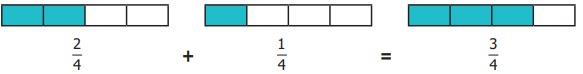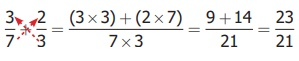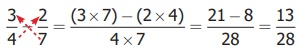Home | | Maths 6th Std | Addition and Subtraction of Unlike Fractions

# Addition and Subtraction of Unlike Fractions

To add or subtract two unlike fractions, first we need to convert them into like fractions.

Addition and Subtraction of Unlike Fractions

Venkat went to buy milk. He bought 1/2 litre first and then he bought 1/4 litre. He wanted to find how much milk he bought altogether? In order to find the total quantity of milk, he has to add 1/2 and 1/4. That is 1/2 + 1/4. To add or subtract two unlike fractions, first we need to convert them into like fractions.

Example 5

The teacher had given the same situation mentioned above and asked two  students Ravi and Arun to solve it. They came out with the  answers for  ½ + ¼.

Solution

Ravi’s way

Common Multiple of 2 and 4 = 4.

Equivalent Fractions of

1/2 = (1 ×2) / (2 ×2) = 2/4

Now, add 1/2 + 1/4 = 2/4 + 1/4

= (2 + 1) / (4 + 4) = 3/8

Therefore Venkat has bought 3/8 litres of milk.

Arun’s way

Common Multiple of 2 and 4 = 4.

Equivalent Fractions of

1/2 = (1 ×2) / (2 ×2) = 2 /4

Now, add 1/2+ 1/4 = 2/4 +1/4

= (2 + 1) / 4 = 3/4

Therefore Venkat has bought 3/4 litres of milk.

The teacher concludes that Arun’s way is correct. This can be verified by the following diagram.In the above illustration note that while adding two like fractions the total number of parts (denominator) remains the same and the two shaded parts (numerator) are added.

Example 6

Solution

These are unlike fractions, aren’t they ? So first we need to convert them into like fractions? Is it possible? Yes, always. How do we do so? The common multiple of 3 and 5 is 15. Hence, we find the equivalent fractions of 2/3 and 3/5 with denominator 15.2/3 = (2 × 5) / (3 × 5) = 10 / 15

3/5 = (3 × 3) / (5 × 3) = 9 / 15

2/3 + 3/5 = 10/15 + 9/15 = 19 / 15

In the above example, the common denominator is 15 (3×5). Now we observe that the numerator and denominator of the first fraction is multiplied by 5 which is the denominator of the second fraction. In the same way, the second fraction is multiplied by 3 which is the denominator of the first fraction. Now in finding the numerator of both the like fractions, we need to multiply the numerator of the first fraction by 5 and the numerator of the second by 3. In the denominator, 3×5 and 5×3 are of course the same. Thus, the technique of finding the like fraction is called Cross Multiplication technique.Example 7

Simplify:Solution

By Cross Multiplication technique,Vani has 3/4  litre of water in her bottle. She drank 1/2 litre of it. How much water is  left in the bottle? To find the amount of water remaining in her bottle, the amount of water consumed by her must be subtracted from the amount of water she had initially. That is 3/4 1/2 . This is solved in the following example.

Example 8

Simplify: 3/4 – 1/2

Solution

Common multiple of 2 and 4 is 4Therefore, Vani has1/4 amount of water in the bottle. This can be verified by the following diagram.Example 9

Find the difference between 3/4 and 2/7.

Solution

To find the difference, first we should know, which is bigger between the two given fractions? How do we find out?

We know that comparing 'like fractions' is easy, but these are unlike fractions.

So, let us convert them into 'like fractions' and compare. Again, we use common multiple 28 (4×7) to get equivalent fractions of 3/4 and 2/7 as 21/28 and 8/28. Here, 21/28 > 8/28 and hence  3/4 > 2/7.

Therfore, 3/4 − 2/7 = 21/28 − 8/28 = 13/28.

This can also be done by Cross Multiplication technique as= [21 – 8] / 28 = 13/28 .

Try these

i) 2/3 + 5/7

[ (2 × 7) + (5 × 3) ] / [3 × 7]

[14 + 15] / 21 =  29/31

ii) 3/5 – 3/8

[24 – 15] / [5 × 8] = 9/40

Activity

Using the given fractions 1/5, 1/6, 1/10 , 1/15 , 2/15 , 4/15 , 1/30 , 7/30 and 9/30  fill in the missing ones in the given 3×3 square in such a way that the addition of fractions through rows, columns and diagonals give the same total 1/2 .1/30 + 1/6 = [1 + 5]/30 = 6/30 = 1/5

1/2 – 6/30 = [15 – 6]/30 = 9/30 = 3/10

2/15 – 3/10 = [4 + 9]/30 = 13/30

1/2 – 13/30 = [15 – 13]/30 = 2/30 = 1/15

1/6 + 1/15 = [5 + 2]/30 = 7/30

1/2 – 7/30 = [15 – 7]/30 = 8/30 = 4/15

4/15 + 1/30 = [8 + 1]/30 = 9/30 = 3/10

1/2 – 9/30 = [15 – 9]/30 = 6/30 = 1/5

4/15 + 2/15 = 6/15 = 2/5

1/2 – 2/5 = [5 – 4]/10 = 1/10

1/2 – 4/15 = [15 – 8]/30 = 7/30Tags : Fractions | Term 3 Chapter 1 | 6th Maths , 6th Maths : Term 3 Unit 1 : Fractions
Study Material, Lecturing Notes, Assignment, Reference, Wiki description explanation, brief detail
6th Maths : Term 3 Unit 1 : Fractions : Addition and Subtraction of Unlike Fractions | Fractions | Term 3 Chapter 1 | 6th Maths